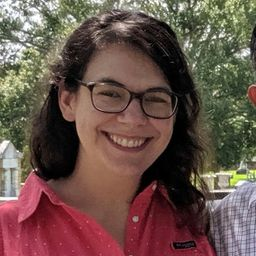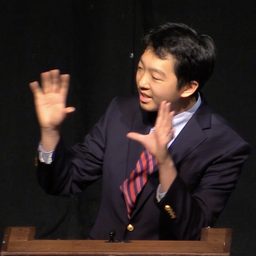# Geometry A Common Core Curriculum

## Educators### Problem 1

COMPLETE THE SENTENCE Two lines that do not intersect and are also not parallel are ________ lines.Margaret F.

### Problem 2

WHICH ONE DOESN’T BELONG? Which angle pair does not belong with the other three? Explain your reasoning.Zachary Z.

### Problem 3

In Exercises $3-6,$ think of each segment in the diagram as part of a line. All the angles are right angles. Which line(s) or plane(s) contain point $B$ and appear to $l$ the description?
line(s) parallel to $\mathrm{CD}$Joseph L.

### Problem 4

In Exercises $3-6,$ think of each segment in the diagram as part of a line. All the angles are right angles. Which line(s) or plane(s) contain point $B$ and appear to $l$ the description?
line(s) perpendicular to CDZachary Z.

### Problem 5

In Exercises $3-6,$ think of each segment in the diagram as part of a line. All the angles are right angles. Which line(s) or plane(s) contain point $B$ and appear to $l$ the description?
line(s) skew to CDJoseph L.

### Problem 6

In Exercises $3-6,$ think of each segment in the diagram as part of a line. All the angles are right angles. Which line(s) or plane(s) contain point $B$ and appear to $l$ the description?
plane(s) parallel to plane CDH

Check back soon!

### Problem 7

In Exercises $7-10,$ use the diagram.
Name a pair of parallel lines.Joseph L.

### Problem 8

In Exercises $7-10,$ use the diagram.
Name a pair of perpendicular lines.Zachary Z.

### Problem 9

In Exercises $7-10,$ use the diagram.
Is $\mathrm{PN} \rceil$ KM? Explain.Joseph L.

### Problem 10

In Exercises $7-10,$ use the diagram.
Is $\mathrm{PR} \perp \mathrm{NP}$ ? Explain.Zachary Z.

### Problem 11

In Exercises $11-14$ , identify all pairs of angles of the given type.
correspondingJoseph L.

### Problem 12

In Exercises $11-14$ , identify all pairs of angles of the given type.
alternate interiorZachary Z.

### Problem 13

In Exercises $11-14$ , identify all pairs of angles of the given type.
alternate exteriorJoseph L.

### Problem 14

In Exercises $11-14$ , identify all pairs of angles of the given type.
consecutive interiorZachary Z.

### Problem 15

USING STRUCTURE Exercises $15-18,$ classify the angle pair as corresponding, alternate interior, alternate exterior, or consecutive interior angles.Joseph L.

### Problem 16

USING STRUCTURE Exercises $15-18,$ classify the angle pair as corresponding, alternate interior, alternate exterior, or consecutive interior angles.
$$<11 \text { and } \angle 13$$Zachary Z.

### Problem 17

USING STRUCTURE Exercises $15-18,$ classify the angle pair as corresponding, alternate interior, alternate exterior, or consecutive interior angles.
$$\angle 6 \text { and } \angle 13$$Joseph L.

### Problem 18

USING STRUCTURE Exercises $15-18,$ classify the angle pair as corresponding, alternate interior, alternate exterior, or consecutive interior angles.
$$\angle 2 \text { and } \angle 11$$Zachary Z.

### Problem 19

ERROR ANALYSIS Exercises 19 and 20 , describe and correct the error in the conditional statement about lines.
If two lines do not intersect, then they are parallel.Margaret F.

### Problem 20

ERROR ANALYSIS Exercises 19 and 20 , describe and correct the error in the conditional statement about lines.
If there is a line and a point not on the line, then there is exactly one line through the point that intersects the given line.Zachary Z.

### Problem 21

MODELING WITH MATHEMATICSe the photo to decide whether the statement is true or false. Explain your reasoning.
a. The plane containing the oor of the tree house is parallel to the ground.
b. The lines containing the railings of the staircase, such as $A B$ , are skew to all lines in the plane containing the ground.Joseph L.

### Problem 22

THOUGHT PROVOKINGf two lines are intersected by a third line, is the third line necessarily a transversal? Justify your answer with a diagram.Zachary Z.

### Problem 23

MATHEMATICAL CONNECTIONSO lines are cut by a transversal. Is it possible for all eight angles formed to have the same measure? Explain your reasoning.Margaret F.

### Problem 24

HOW Do You SEE ITThink of each segment in the gure as part of a line.
a. Which lines are parallel to $\mathrm{NQ}$ ?
b. Which lines intersect $\mathrm{NQ}$ ?
c. Which lines are skew tove?Zachary Z.

### Problem 25

Use the diagram to I nd the measures of all the angles. (Section 2.6)
$$\mathrm{m} \angle 1=76^{\circ}$$Joseph L.

### Problem 26

In Exercises $25-28$ , copy and complete the statement List all possible correct answers.
$\angle \mathrm{BCG}$ andZachary Z.

### Problem 27

In Exercises $25-28$ , copy and complete the statement. List all possible correct answers.
$\angle \mathrm{FCJ}$ and ______ are alternate interior angles.Joseph L.

### Problem 28

In Exercises $25-28$ , copy and complete the statement. List all possible correct answers.
$\angle \mathrm{FCA}$ and

Check back soon!

### Problem 29

MAKING AN ARGUMENT YOur friend claims the uneven parallel bars in gymnastics are not really parallel. She says one is higher than the other, so they cannot be in the same plane. Is she correct? Explain.Margaret F.

### Problem 30

NO QUESTION YETZachary Z.
$\mathrm{m} \angle 2=159^{\circ}$